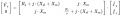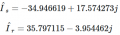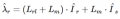# How to calculate rotor flux of the three phase squirrel cage induction motor?

Joined Mar 1, 2016
14
Three phase squirrel cage induction motor with given parameters (stator winding connected in delta)
• nominal power: Pn=22.4kW
• nominal stator voltage: Vsn=230V
• nominal stator current: Isn=39.5A
• nominal stator frequency: fsn=60Hz
• nominal speed: nn=1168min−1
• number of pole pairs: 3
• stator resistance per phase (T equivalent circuit): Rs=0.294Ω
• stator leakage inductance per phase (T equivalent circuit): Lsl=0.00139H
• rotor resistance per phase (T equivalent circuit): Rr=0.156Ω
• rotor leakage inductance per phase (T equivalent circuit): Lrl=0.0007401H
• magnetizing inductance per phase (T equivalent circuit): Lm=0.041H

I have been struggling with calculation of the nominal value of the rotor flux. My idea was that I will use the T equivalent circuit for that purposeand I will use nominal values of the motor quantities i.e. I set the motor operating point into the nominal operation point (nominal slip, nominal stator voltage etc.). Then I calculate phasor of the stator current (Is) and phasor of the rotor current (Ir) according to the below given set of equationsFor the motor parameters mentioned above the Scilab command linsolve gave meBased on the known phasors of the stator and rotor current I used the below given equation for calculation of the phasor of the rotor fluxwhich gives λr=0.5554856−0.0613638j i.e. |λr|=0.5588647V⋅s.

This value seems to me to be too low. So I have doubts regarding the way I have used for its calculation. Unfortunately I don't know any other way for its calculation which I can use for verification. Can anybody tell me whether the applied procedure is correct or not?